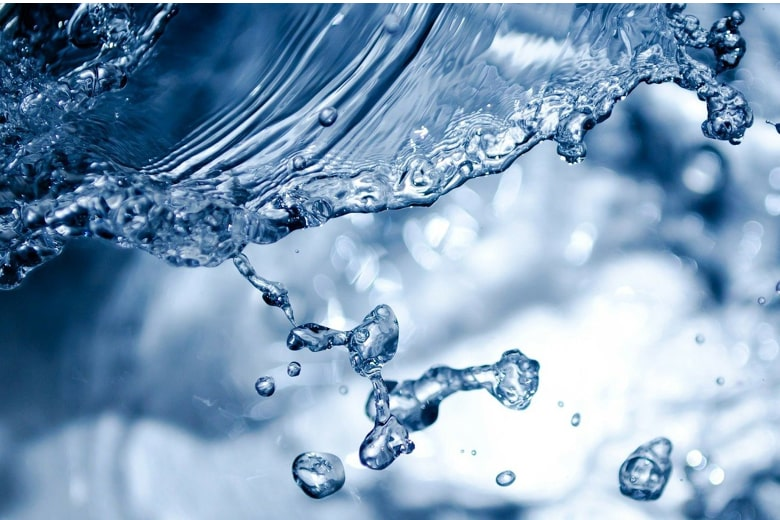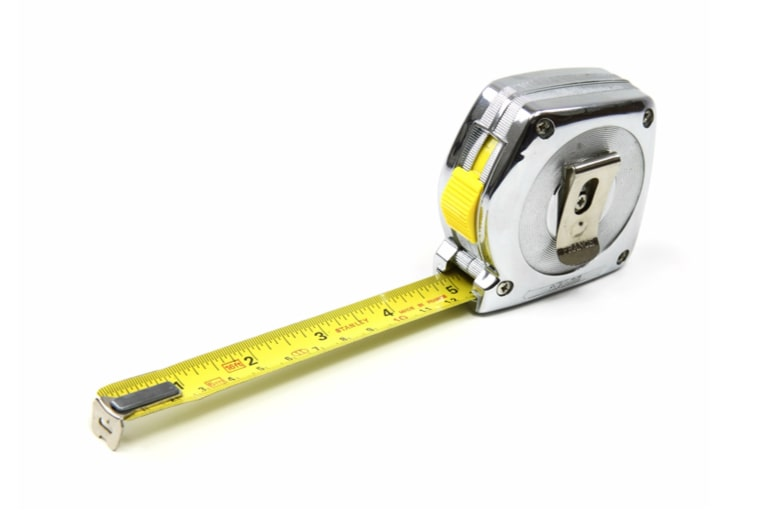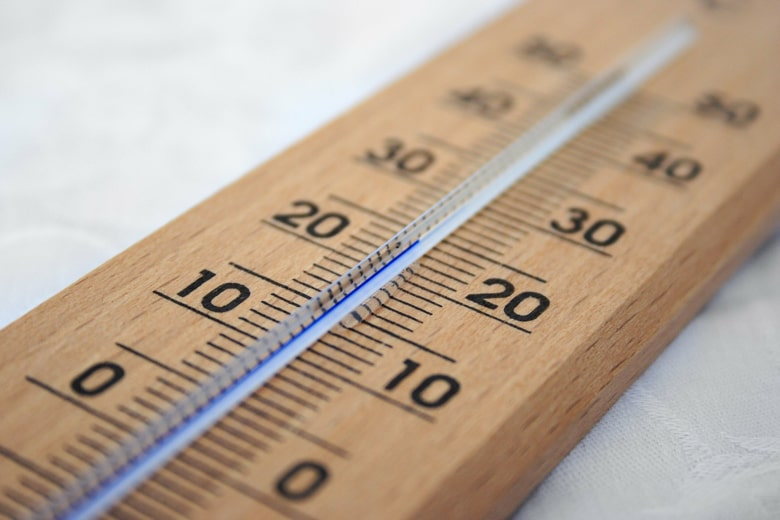# Unit Converters for Instrumentation Engineers

## Our best converters

In this category we will find useful tools for the instrumentation engineer, such as the measurement of Normal, Current and Standard flow or the calculation of the amount of product that leaks through a pipe. We will gradually add more tools to this section. The results of all calculators are exportable in spreadsheet format. If you have any questions or require further clarification, do not hesitate to contact us.### Density Unit Converter Online

This simple and easy online density unit converter let you convert several types of density units.### Volumetric Flow Converter

This simple and easy online flow unit converter let you convert several types of flow units.### Length Converter

This simple and easy online length unit converter let you convert several types of length units.### Pressure Unit Converter

This simple and easy online pressure unit converter let you convert several types of pressure units.### Temperature Unit Converter

This simple and easy online temperature unit converter let you convert several types of temperature units.### Viscosity Converter

This simple and easy online viscosity unit converter let you convert several types of viscosity units.